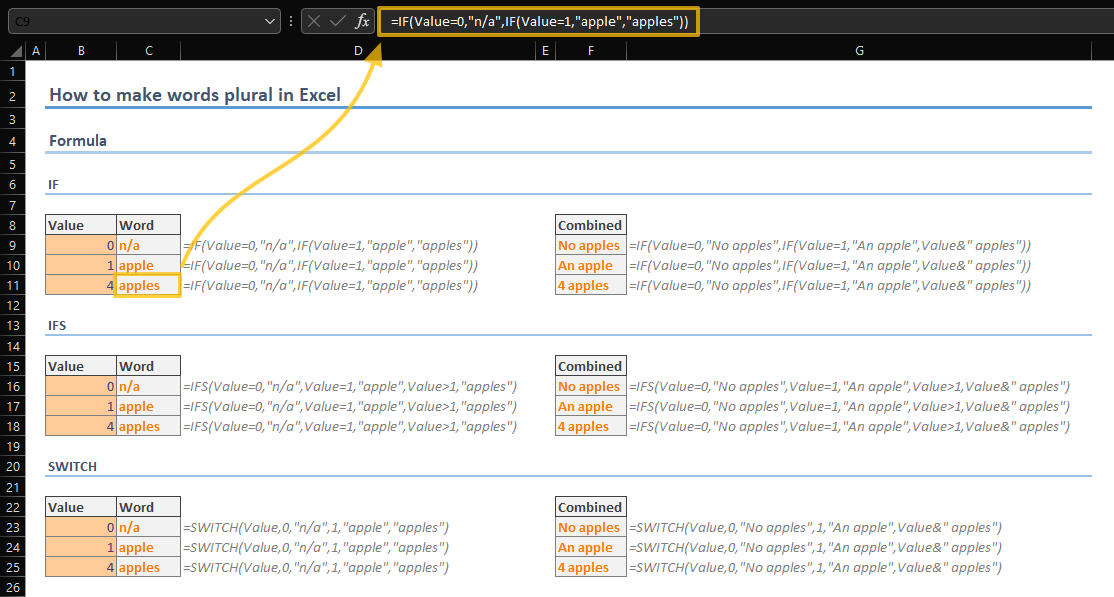Neither words nor grammar is where Excel shines. On the other hand, texts may be handful to inform users what a numeric value means, such as units. Making this type of information changed between singular and plural forms dynamically may increase the end-user experience. In this guide, we’re going to show you How to make words plural in Excel.

## Ways of making words plural in Excel

There are three ways you can use to convert words from singular to plural form.

1. The obvious method is using formulas. Either an IF logic or lookup functions with a predefined table can return the corresponding form into a cell.
2. The other method is Custom Number Formatting which allows you to display a word, possibly the unit of the number, in the same cell of the value without changing the cell value. Thus, you can use the numeric value in other formulas if needed.
3. The last method is VBA. A simple macro can alter any cell's value and make a word plural or singular.

### Formula way

#### Conditions

The logic is simple. If the value is greater than 1, use the plural form. Otherwise use the singular. Of course, you may want to add rules for 0 and negative amounts. However, these types of rule additions will not change the syntax much.

In our example, we have samples for IF, IFS and SWITCH formulas. For the base greater than 1 rule, the good old IF function is more than enough. For more rules, consider using the IFS or the SWITCH function to not deal with nested formulas.

Here are the syntaxes for the formulas which cover 0 values as well.

=IF(<value>=0,<zero-items>,IF(<value>=1,<singular form>,<plural form>))

=IFS(<value>=0,<zero-items>,<value>=1,<singular form>,Value>1,<plural form>)

=SWITCH(Value,0,<zero-items>,1,<singular form>,<plural form>)#### Lookup

Unfortunately, making a word plural is not simple as adding "-s" suffix. If you must deal with multiple words with different plural formations, lookup formulas may ease your life.

To use the lookup formulas, you need a lookup table which should contain both singular and plural forms of the words.

 Singular Plural grape grapes peach peaches blueberry blueberries

You can choose between legacy VLOOKUP, advanced INDEX-MATCH or the newest and the most versatile XLOOKUP functions, to return correct word for your values. While the IF functions determine the column for either singular or plural, the main functions find the matching word.

=VLOOKUP(<word>,<lookup table>,IF(<value>=1,1,2),0)

=INDEX(<lookup table>,MATCH(<word>,<lookup table's singular column>,0),IF(<value>=1,1,2))

=XLOOKUP(<word>,<lookup table's singular column>,IF(<value>=1,<lookup table's singular column>,<lookup table's plural columns>))### Number Formatting

Excel's Number Formatting feature allows us a fancier way to display singular and plural names based on a cell's value. The main advantage of this method is that it saves you from helper cells or columns to write your formulas and display the word. You can keep the logic in the cell itself without sacrificing its numerical value.

A number formatting like a currency or percentage does not alter the value of the cell. It's just cosmetics.The downside of this method is the 3-condition limit. You cannot nest multiple rules like the formulas.

Follow the steps to apply this kind of number formatting.

1. Select your cells which contain the values
2. Open Format Cells dialog by either pressing Ctrl + 1 or right-click and selecting Format Cells.
3. In the Format Cells dialog, make sur ethe Number tab is active.
4. Select the Custom under Category
5. Type the rule according to your needs. (Ours: [=1]0 "apple";[>1]0 "apples";"No apples" )
6. Click OK button to apply the formatting.The custom number formatting rule can contain up-to 3 conditions including the else­ condition. The logical expression should be defined between square brackets ([]). Our rule can be deciphered as;

• If the value is equal to 1, show the value (0 represents a numeric value) with the string "apple"
• If the value is greater than 1, show the value with the string "apples"
• Any other situation, display "No apples" string without displaying the value. This covers zero and negative values.

Do not hesitate to jump on Number Formatting in Excel - All You Need to Know article to learn more about the number formatting.